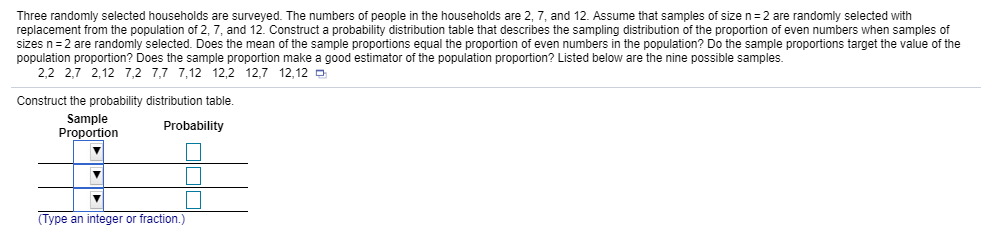# Three randomly selected households are surveyed. The numbers of people in the households are 2 7, and 12 Assume that samples of size n=2 are randomly selected with replacement from the population of 2, 7, and 12. Construct a probability distribution table that describes the sampling distribution of the proportion of even numbers when samples of sizes n = 2 are randomly selected. Does he mean o he sample proportions equal he proportion of even number in he population? Do the sample opo 10 s a ett evalue of the population proportion? Does the sample proportion make a good estimator of the population proportion? Listed below are the nine possible samples. 2,2 2,7 2,12 7,2 7,7 7,12 12,2 12,7 12,12 Construct the probability distribution table. Sample ProportionProbability Type an integer or fraction.)

Question
4366 viewshelp_outlineImage TranscriptioncloseThree randomly selected households are surveyed. The numbers of people in the households are 2 7, and 12 Assume that samples of size n=2 are randomly selected with replacement from the population of 2, 7, and 12. Construct a probability distribution table that describes the sampling distribution of the proportion of even numbers when samples of sizes n = 2 are randomly selected. Does he mean o he sample proportions equal he proportion of even number in he population? Do the sample opo 10 s a ett evalue of the population proportion? Does the sample proportion make a good estimator of the population proportion? Listed below are the nine possible samples. 2,2 2,7 2,12 7,2 7,7 7,12 12,2 12,7 12,12 Construct the probability distribution table. Sample ProportionProbability Type an integer or fraction.) fullscreen

### Want to see this answer and more?

Step-by-step answers are written by subject experts who are available 24/7. Questions are typically answered within 1 hour.*